Скачать презентацию An Analysis for Troubled Assets Reverse Auction Saeed

• Количество слайдов: 38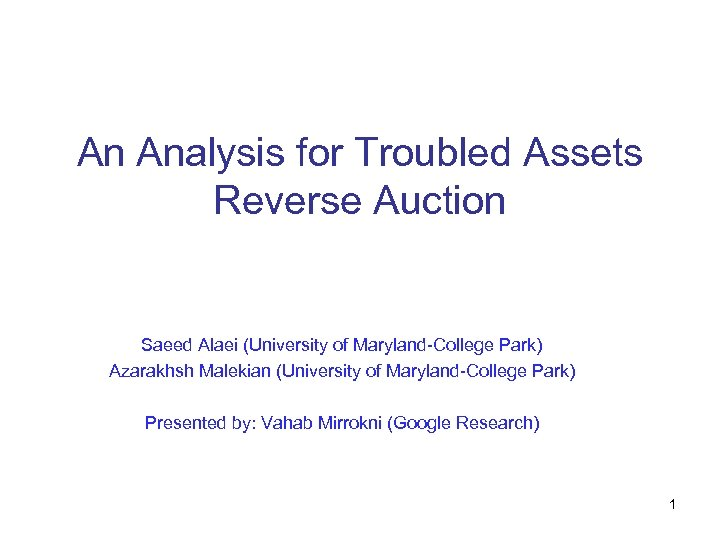An Analysis for Troubled Assets Reverse Auction Saeed Alaei (University of Maryland-College Park) Azarakhsh Malekian (University of Maryland-College Park) Presented by: Vahab Mirrokni (Google Research) 1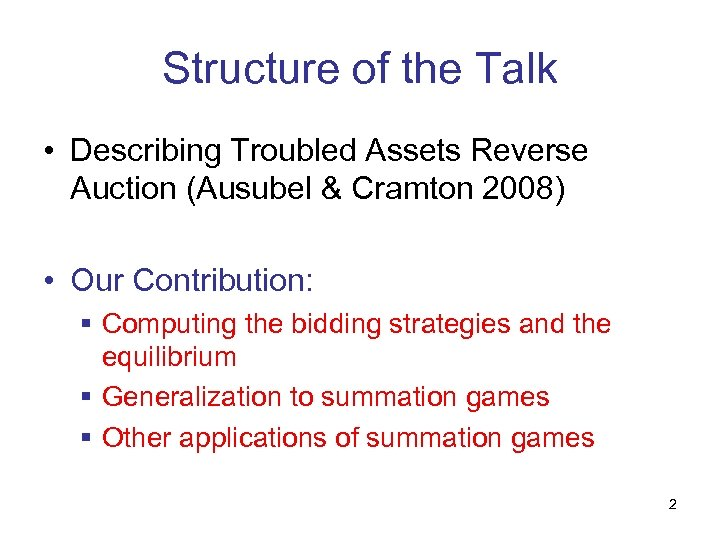Structure of the Talk • Describing Troubled Assets Reverse Auction (Ausubel & Cramton 2008) • Our Contribution: § Computing the bidding strategies and the equilibrium § Generalization to summation games § Other applications of summation games 2Economic Crisis • In a normal economy, banks sell their illiquid assets when they need more liquidity. • In the crisis, the need for liquidity goes up • Financial companies try to turn their illiquid assets to liquidity • Supply of illiquid assets in the market increases, so market clearing prices go down • Companies lose as the result of the prices of their assets going down. 3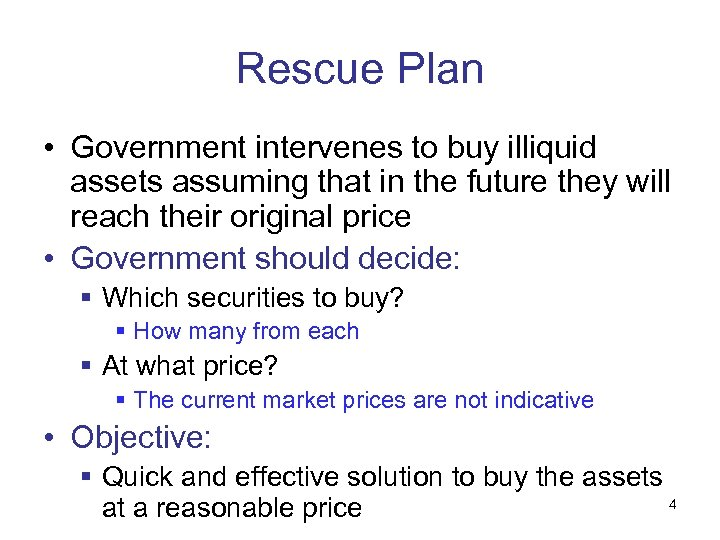Rescue Plan • Government intervenes to buy illiquid assets assuming that in the future they will reach their original price • Government should decide: § Which securities to buy? § How many from each § At what price? § The current market prices are not indicative • Objective: § Quick and effective solution to buy the assets 4 at a reasonable price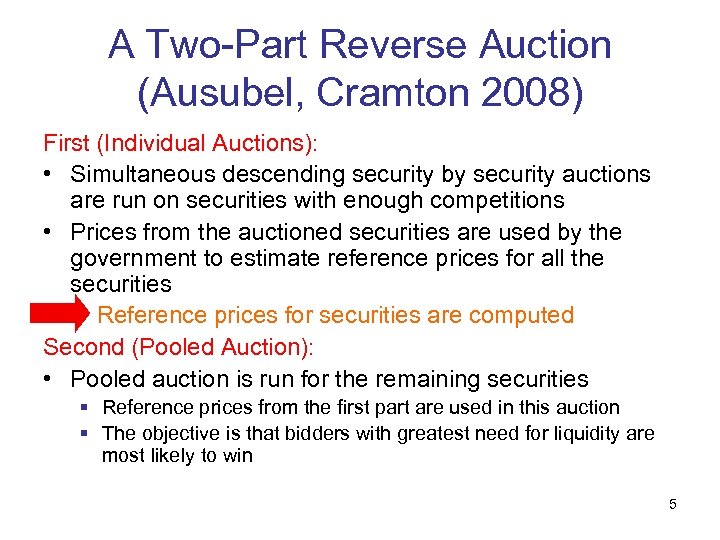A Two-Part Reverse Auction (Ausubel, Cramton 2008) First (Individual Auctions): • Simultaneous descending security by security auctions are run on securities with enough competitions • Prices from the auctioned securities are used by the government to estimate reference prices for all the securities Reference prices for securities are computed Second (Pooled Auction): • Pooled auction is run for the remaining securities § Reference prices from the first part are used in this auction § The objective is that bidders with greatest need for liquidity are most likely to win 5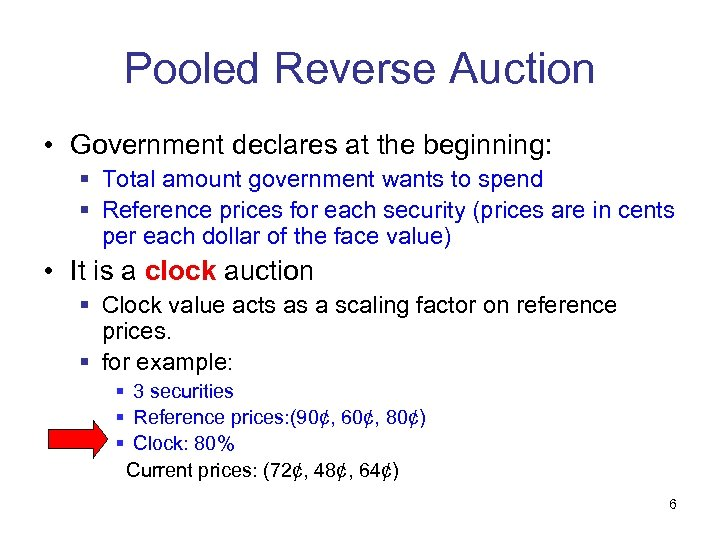Pooled Reverse Auction • Government declares at the beginning: § Total amount government wants to spend § Reference prices for each security (prices are in cents per each dollar of the face value) • It is a clock auction § Clock value acts as a scaling factor on reference prices. § for example: § 3 securities § Reference prices: (90¢, 60¢, 80¢) § Clock: 80% Current prices: (72¢, 48¢, 64¢) 6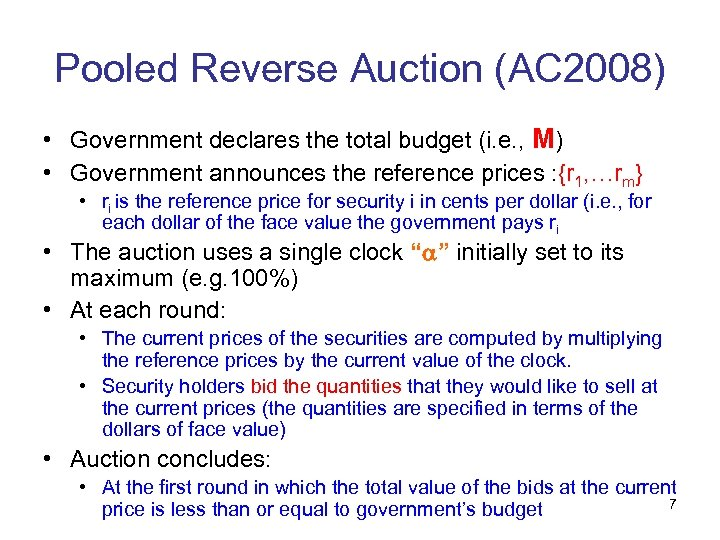Pooled Reverse Auction (AC 2008) • Government declares the total budget (i. e. , M) • Government announces the reference prices : {r 1, …rm} • ri is the reference price for security i in cents per dollar (i. e. , for each dollar of the face value the government pays ri • The auction uses a single clock “ ” initially set to its maximum (e. g. 100%) • At each round: • The current prices of the securities are computed by multiplying the reference prices by the current value of the clock. • Security holders bid the quantities that they would like to sell at the current prices (the quantities are specified in terms of the dollars of face value) • Auction concludes: • At the first round in which the total value of the bids at the current 7 price is less than or equal to government’s budget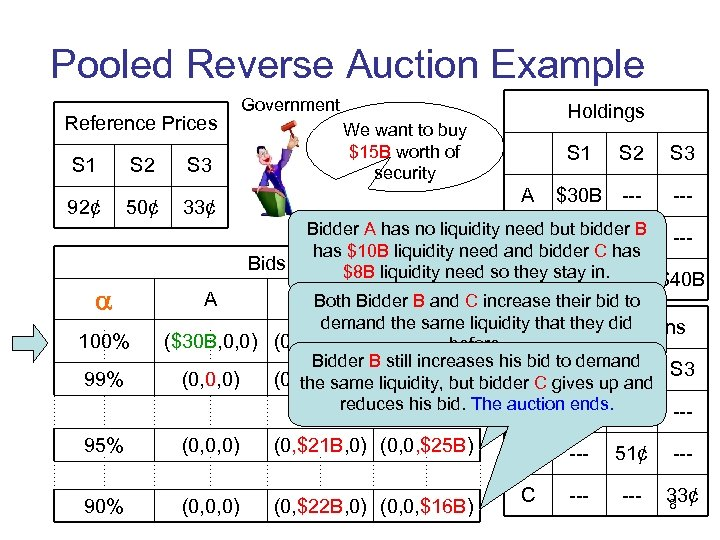Pooled Reverse Auction Example Reference Prices S 1 S 2 50¢ 33¢ 100% 99% A S 1 A Bids Holdings We want to buy \$15 B worth of security S 3 92¢ Government S 2 S 3 \$30 B --- Bidder A has no liquidity need but bidder B B --- \$35 B --has \$10 B liquidity need and bidder C has \$8 B liquidity need so they stay in. --- \$40 B C --- B Bidder B and C increase their bid to C Both demand the same liquidity that they did Private Valuations (\$30 B, 0, 0) (0, \$20 B, 0) (0, 0, \$40 B) before. Bidder B still increases his bid to demand S 1 (0, 0, 0) (0, \$20 B, 0) liquidity, but bidder C gives up. S 2 S 3 the same (0, 0, \$24 B) and reduces his bid. The auction 91¢ --ends. A 95% (0, 0, 0) (0, \$21 B, 0) (0, 0, \$25 B) 90% (0, 0, 0) (0, \$22 B, 0) (0, 0, \$16 B) --- B --- 51¢ --- C --- 33¢ 8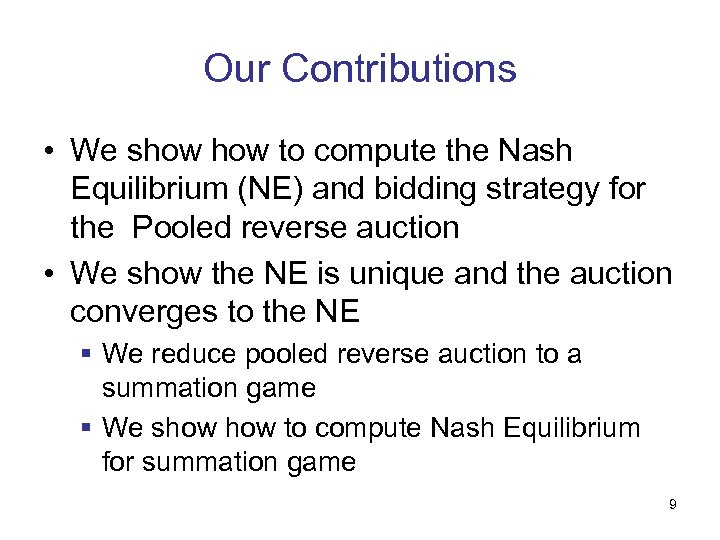Our Contributions • We show to compute the Nash Equilibrium (NE) and bidding strategy for the Pooled reverse auction • We show the NE is unique and the auction converges to the NE § We reduce pooled reverse auction to a summation game § We show to compute Nash Equilibrium for summation game 9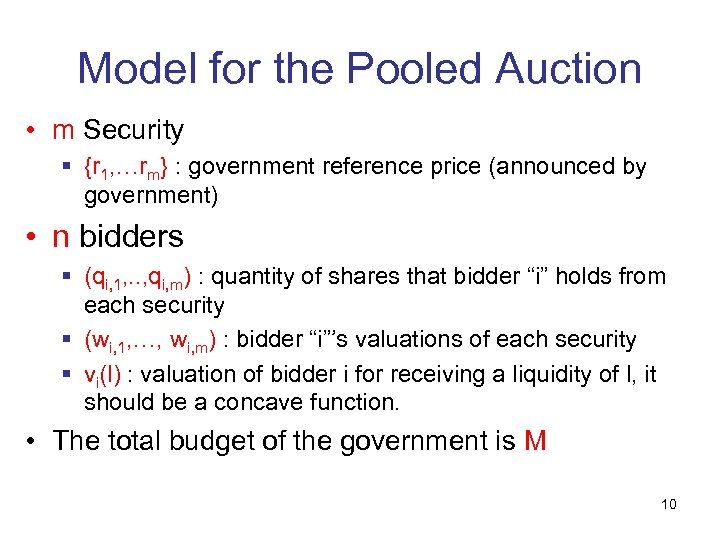Model for the Pooled Auction • m Security § {r 1, …rm} : government reference price (announced by government) • n bidders § (qi, 1, . . , qi, m) : quantity of shares that bidder “i” holds from each security § (wi, 1, …, wi, m) : bidder “i”’s valuations of each security § vi(l) : valuation of bidder i for receiving a liquidity of l, it should be a concave function. • The total budget of the government is M 10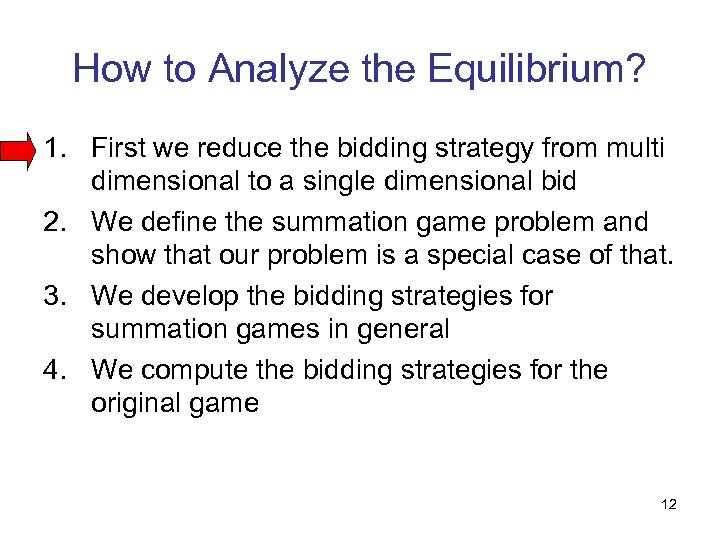How to Analyze the Equilibrium? 1. First we reduce the bidding strategy from multi dimensional to a single dimensional bid 2. We define the summation game problem and show that our problem is a special case of that. 3. We develop the bidding strategies for summation games in general 4. We compute the bidding strategies for the original game 12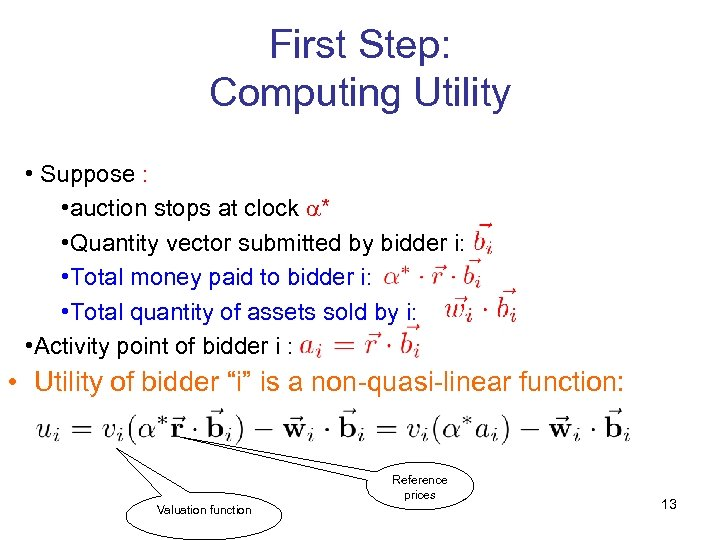First Step: Computing Utility • Suppose : • auction stops at clock * • Quantity vector submitted by bidder i: • Total money paid to bidder i: • Total quantity of assets sold by i: • Activity point of bidder i : • Utility of bidder “i” is a non-quasi-linear function: Reference prices Valuation function 13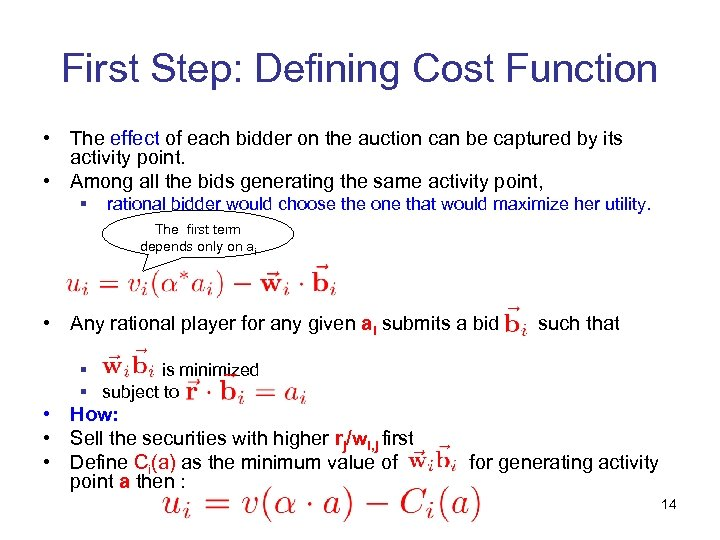First Step: Defining Cost Function • The effect of each bidder on the auction can be captured by its activity point. • Among all the bids generating the same activity point, § rational bidder would choose the one that would maximize her utility. The first term depends only on ai • Any rational player for any given ai submits a bid such that § is minimized § subject to • How: • Sell the securities with higher rj/wi, j first • Define Ci(a) as the minimum value of point a then : for generating activity 14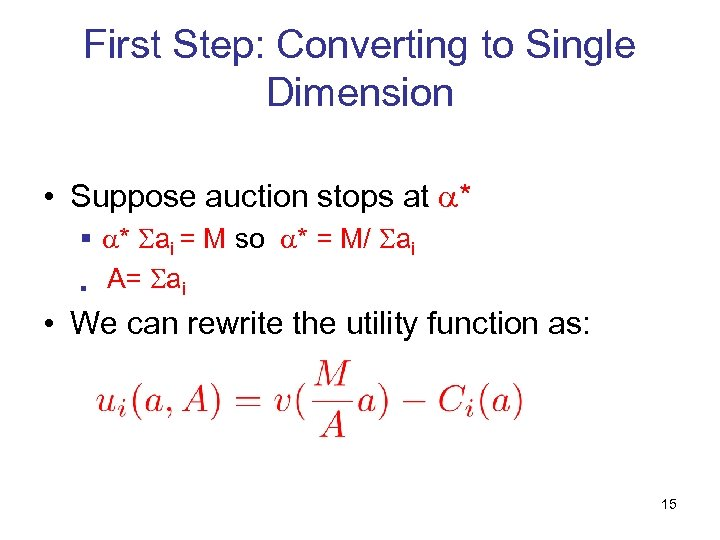First Step: Converting to Single Dimension • Suppose auction stops at * § * ai = M so * = M/ ai § A= ai • We can rewrite the utility function as: 15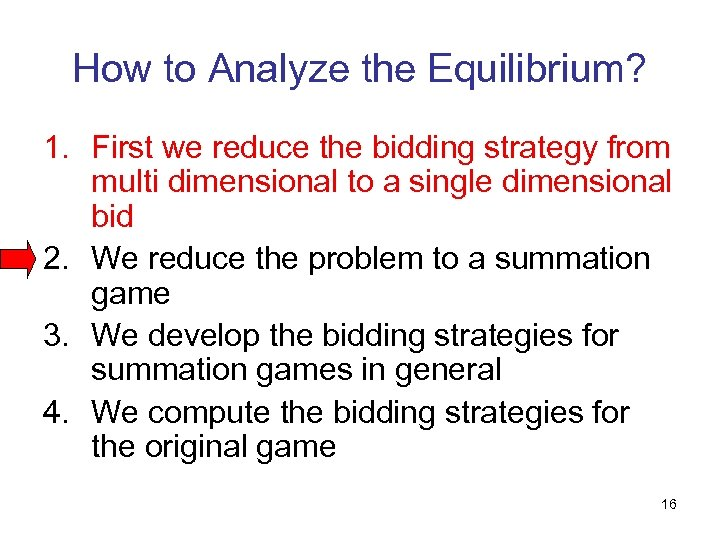How to Analyze the Equilibrium? 1. First we reduce the bidding strategy from multi dimensional to a single dimensional bid 2. We reduce the problem to a summation game 3. We develop the bidding strategies for summation games in general 4. We compute the bidding strategies for the original game 16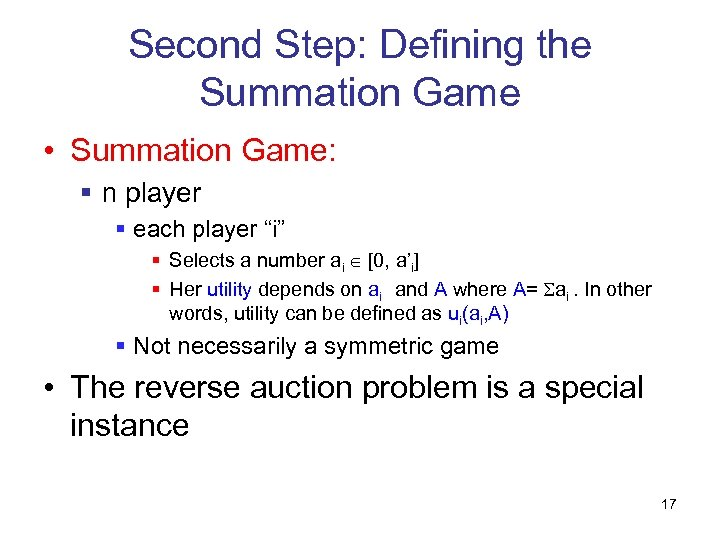Second Step: Defining the Summation Game • Summation Game: § n player § each player “i” § Selects a number ai [0, a’i] § Her utility depends on ai and A where A= ai. In other words, utility can be defined as ui(ai, A) § Not necessarily a symmetric game • The reverse auction problem is a special instance 17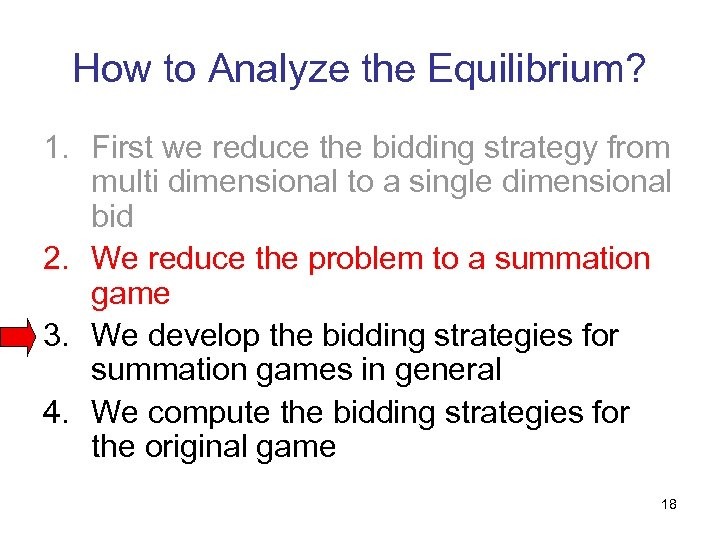How to Analyze the Equilibrium? 1. First we reduce the bidding strategy from multi dimensional to a single dimensional bid 2. We reduce the problem to a summation game 3. We develop the bidding strategies for summation games in general 4. We compute the bidding strategies for the original game 18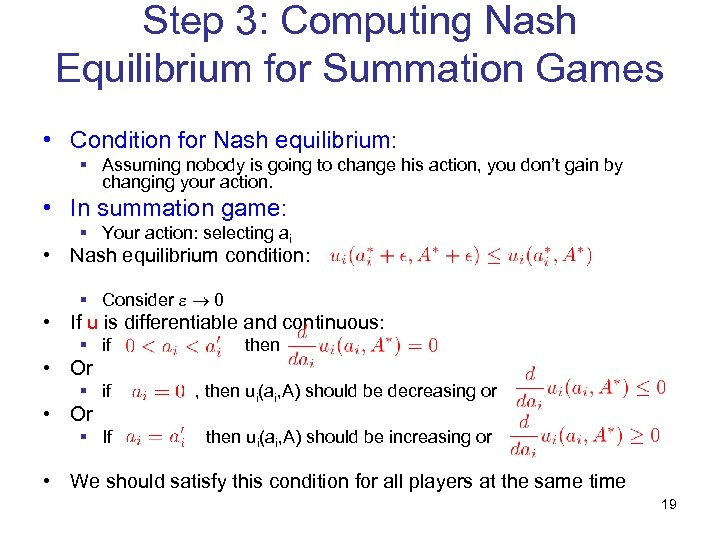Step 3: Computing Nash Equilibrium for Summation Games • Condition for Nash equilibrium: § Assuming nobody is going to change his action, you don’t gain by changing your action. • In summation game: § Your action: selecting ai • Nash equilibrium condition: § Consider 0 • If u is differentiable and continuous: § if then • Or § if • Or § If , then ui(ai, A) should be decreasing or then ui(ai, A) should be increasing or • We should satisfy this condition for all players at the same time 19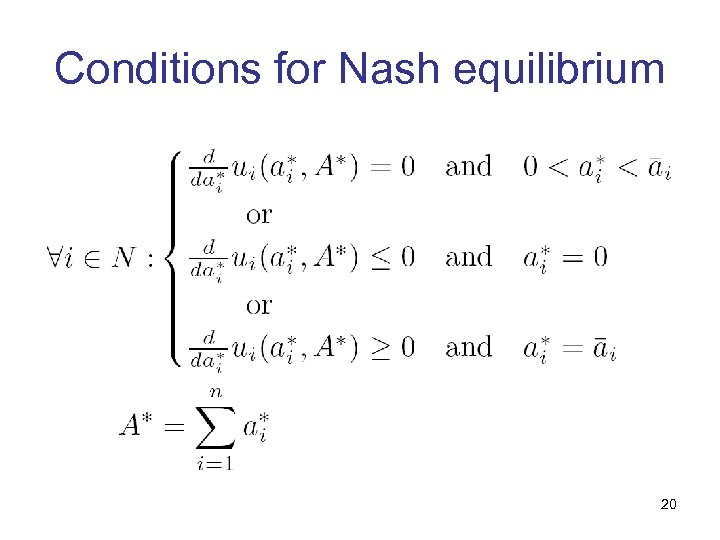Conditions for Nash equilibrium 20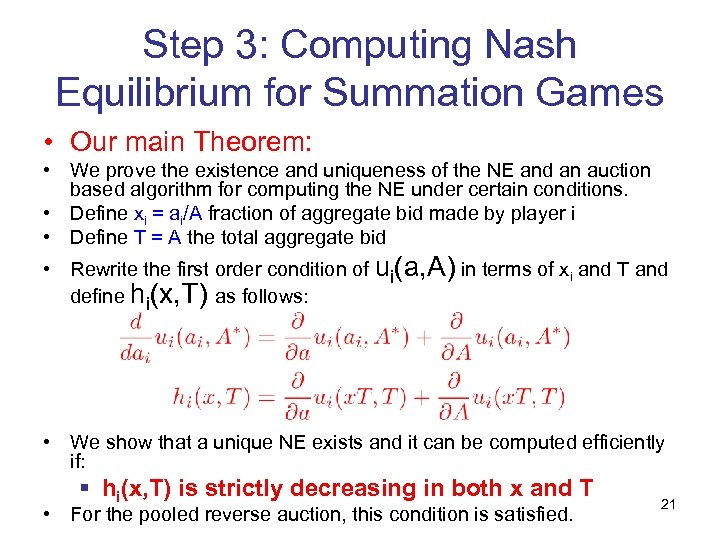Step 3: Computing Nash Equilibrium for Summation Games • Our main Theorem: • We prove the existence and uniqueness of the NE and an auction based algorithm for computing the NE under certain conditions. • Define xi = ai/A fraction of aggregate bid made by player i • Define T = A the total aggregate bid • Rewrite the first order condition of ui(a, A) in terms of xi and T and define hi(x, T) as follows: • We show that a unique NE exists and it can be computed efficiently if: § hi(x, T) is strictly decreasing in both x and T • For the pooled reverse auction, this condition is satisfied. 21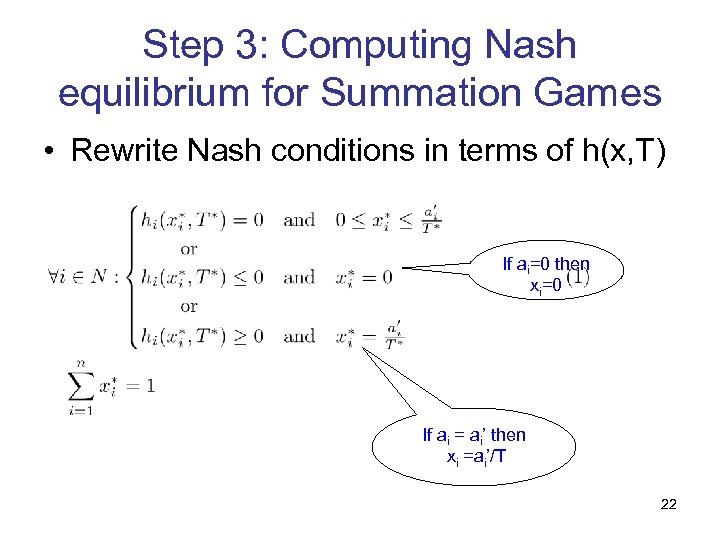Step 3: Computing Nash equilibrium for Summation Games • Rewrite Nash conditions in terms of h(x, T) If ai=0 then xi=0 If ai = ai’ then xi =ai’/T 22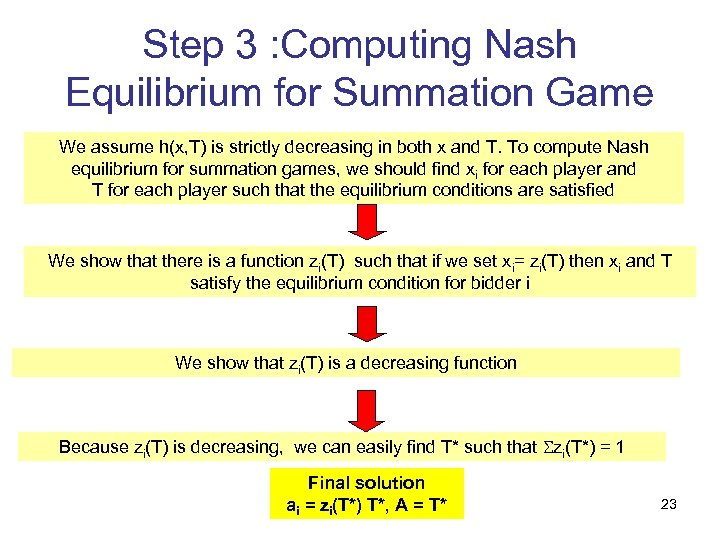Step 3 : Computing Nash Equilibrium for Summation Game We assume h(x, T) is strictly decreasing in both x and T. To compute Nash equilibrium for summation games, we should find xi for each player and T for each player such that the equilibrium conditions are satisfied We show that there is a function zi(T) such that if we set xi= zi(T) then xi and T satisfy the equilibrium condition for bidder i We show that zi(T) is a decreasing function Because zi(T) is decreasing, we can easily find T* such that zi(T*) = 1 Final solution ai = zi(T*) T*, A = T* 23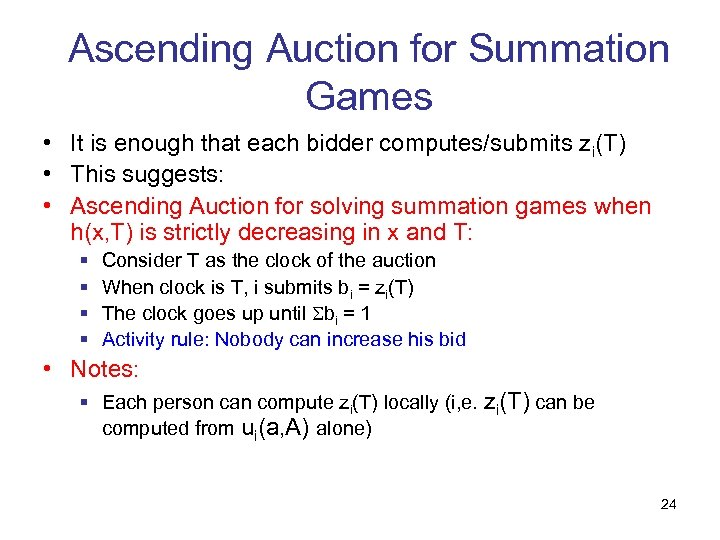Ascending Auction for Summation Games • It is enough that each bidder computes/submits zi(T) • This suggests: • Ascending Auction for solving summation games when h(x, T) is strictly decreasing in x and T: § § Consider T as the clock of the auction When clock is T, i submits bi = zi(T) The clock goes up until bi = 1 Activity rule: Nobody can increase his bid • Notes: § Each person can compute zi(T) locally (i, e. zi(T) can be computed from ui(a, A) alone) 24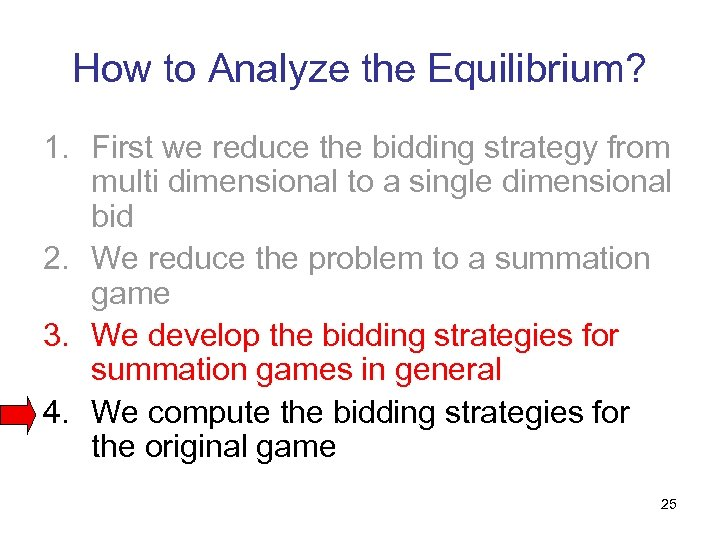How to Analyze the Equilibrium? 1. First we reduce the bidding strategy from multi dimensional to a single dimensional bid 2. We reduce the problem to a summation game 3. We develop the bidding strategies for summation games in general 4. We compute the bidding strategies for the original game 25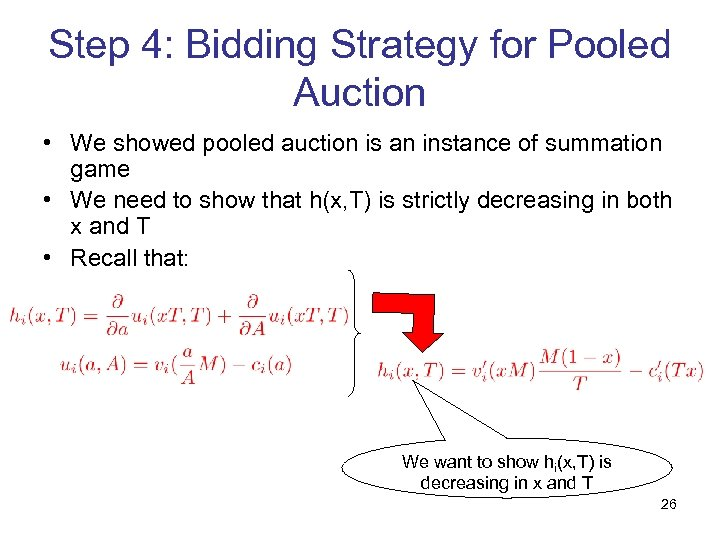Step 4: Bidding Strategy for Pooled Auction • We showed pooled auction is an instance of summation game • We need to show that h(x, T) is strictly decreasing in both x and T • Recall that: We want to show hi(x, T) is decreasing in x and T 26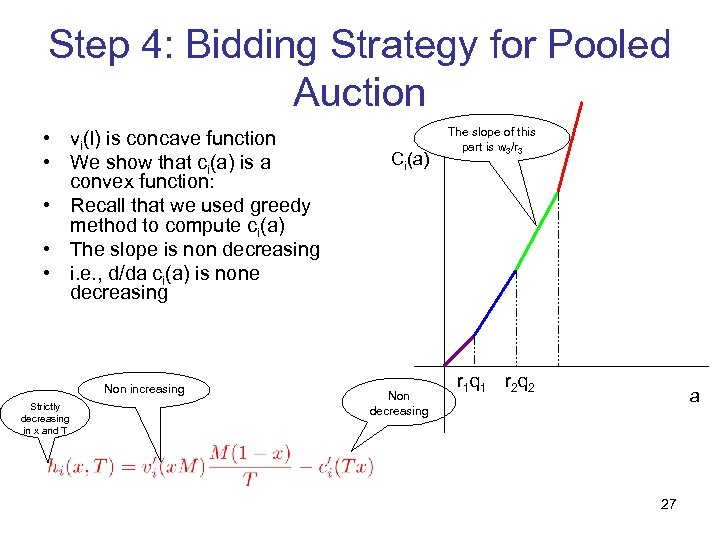Step 4: Bidding Strategy for Pooled Auction • vi(l) is concave function • We show that ci(a) is a convex function: • Recall that we used greedy method to compute ci(a) • The slope is non decreasing • i. e. , d/da ci(a) is none decreasing Non increasing Strictly decreasing in x and T Ci(a) Non decreasing The slope of this part is w 3/r 3 r 1 q 1 r 2 q 2 a 27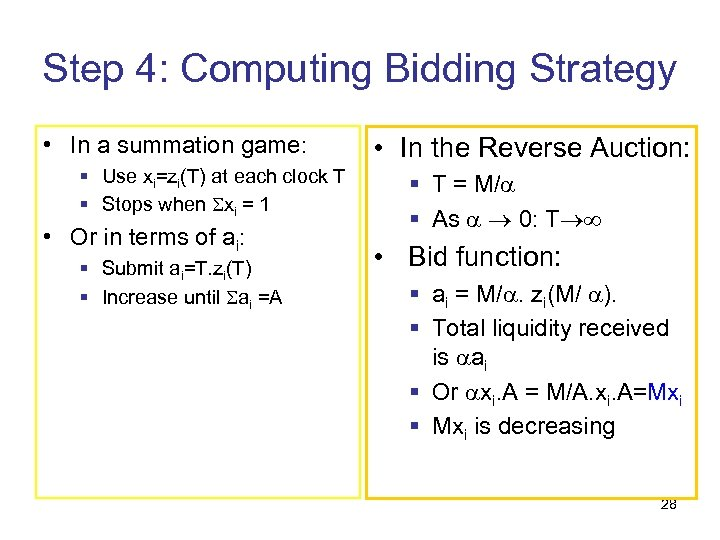Step 4: Computing Bidding Strategy • In a summation game: § Use xi=zi(T) at each clock T § Stops when xi = 1 • Or in terms of ai: § Submit ai=T. zi(T) § Increase until ai =A • In the Reverse Auction: § T = M/ § As 0: T • Bid function: § ai = M/. zi(M/ ). § Total liquidity received is ai § Or xi. A = M/A. xi. A=Mxi § Mxi is decreasing 28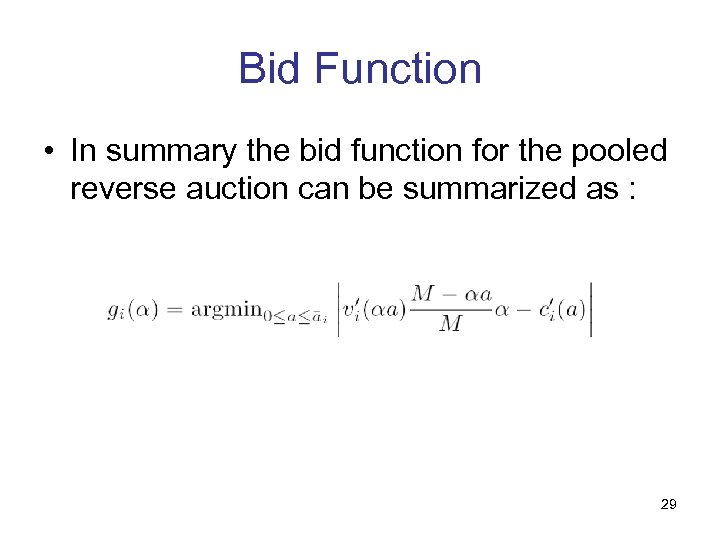Bid Function • In summary the bid function for the pooled reverse auction can be summarized as : 29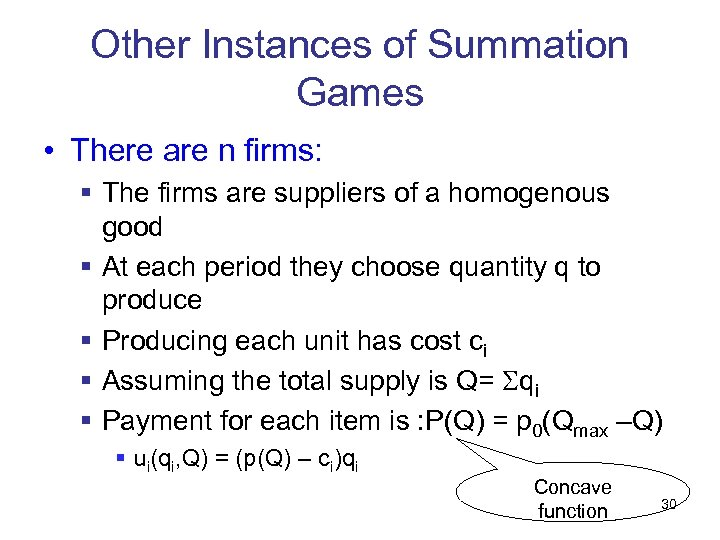Other Instances of Summation Games • There are n firms: § The firms are suppliers of a homogenous good § At each period they choose quantity q to produce § Producing each unit has cost ci § Assuming the total supply is Q= qi § Payment for each item is : P(Q) = p 0(Qmax –Q) § ui(qi, Q) = (p(Q) – ci)qi Concave function 30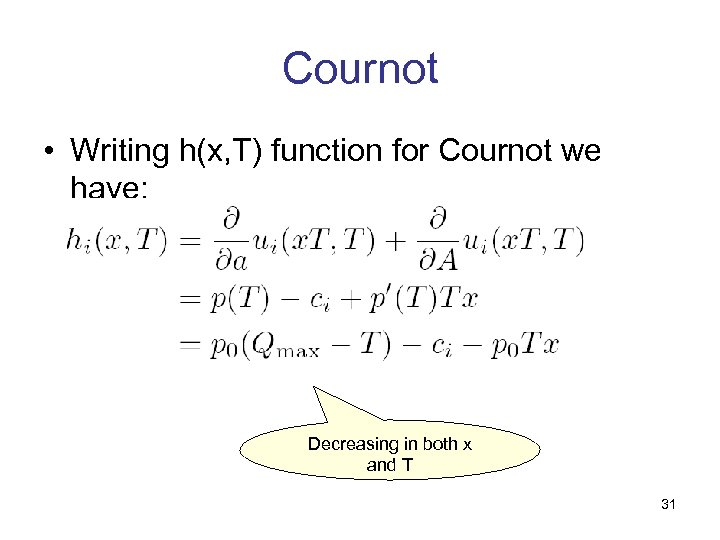Cournot • Writing h(x, T) function for Cournot we have: Decreasing in both x and T 31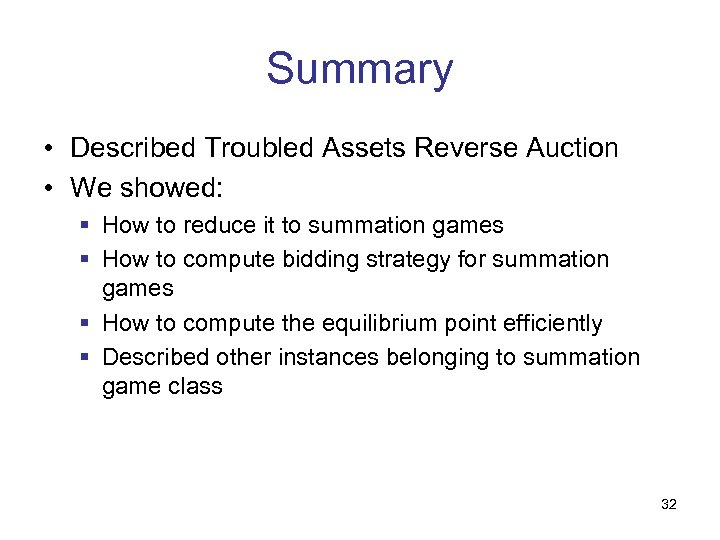Summary • Described Troubled Assets Reverse Auction • We showed: § How to reduce it to summation games § How to compute bidding strategy for summation games § How to compute the equilibrium point efficiently § Described other instances belonging to summation game class 32Questions? 33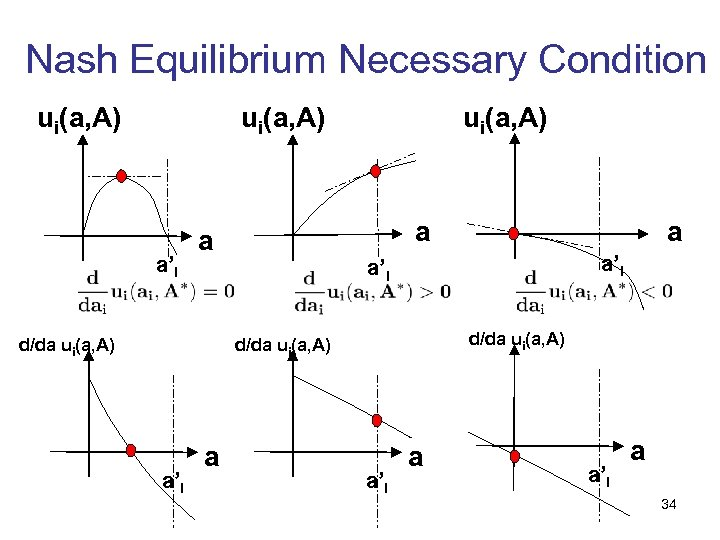Nash Equilibrium Necessary Condition ui(a, A) a’i a a d/da ui(a, A) a’i a a’i a 34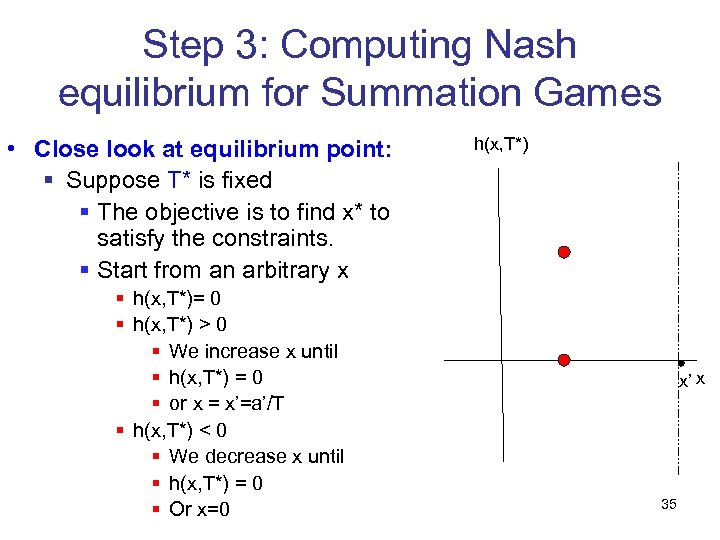Step 3: Computing Nash equilibrium for Summation Games • Close look at equilibrium point: § Suppose T* is fixed § The objective is to find x* to satisfy the constraints. § Start from an arbitrary x § h(x, T*)= 0 § h(x, T*) > 0 § We increase x until § h(x, T*) = 0 § or x = x’=a’/T § h(x, T*) < 0 § We decrease x until § h(x, T*) = 0 § Or x=0 h(x, T*) x’ x 35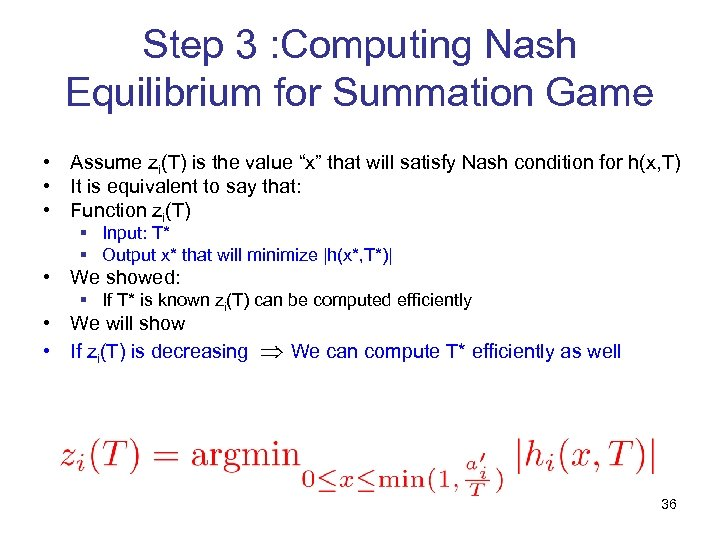Step 3 : Computing Nash Equilibrium for Summation Game • Assume zi(T) is the value “x” that will satisfy Nash condition for h(x, T) • It is equivalent to say that: • Function zi(T) § Input: T* § Output x* that will minimize |h(x*, T*)| • We showed: § If T* is known zi(T) can be computed efficiently • We will show • If zi(T) is decreasing We can compute T* efficiently as well 36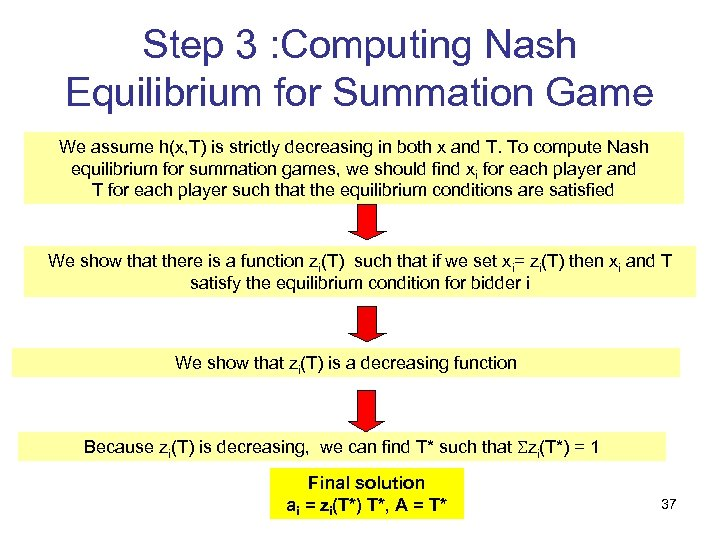Step 3 : Computing Nash Equilibrium for Summation Game We assume h(x, T) is strictly decreasing in both x and T. To compute Nash equilibrium for summation games, we should find xi for each player and T for each player such that the equilibrium conditions are satisfied We show that there is a function zi(T) such that if we set xi= zi(T) then xi and T satisfy the equilibrium condition for bidder i We show that zi(T) is a decreasing function Because zi(T) is decreasing, we can find T* such that zi(T*) = 1 Final solution ai = zi(T*) T*, A = T* 37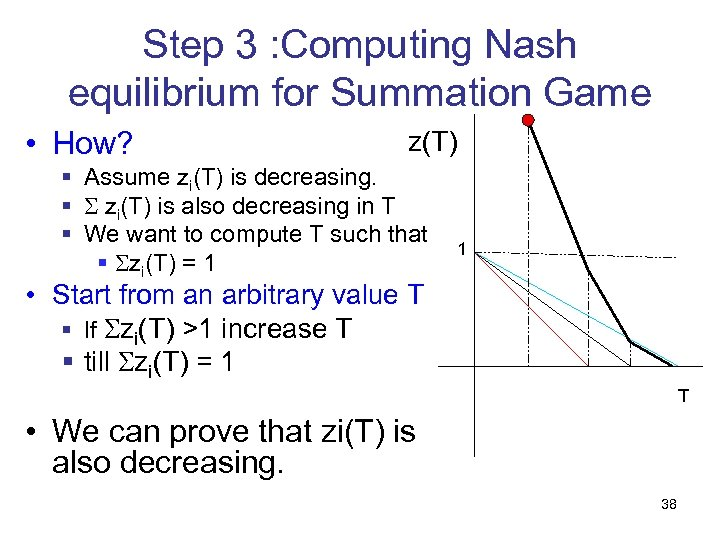Step 3 : Computing Nash equilibrium for Summation Game • How? z(T) § Assume zi(T) is decreasing. § zi(T) is also decreasing in T § We want to compute T such that § zi(T) = 1 1 • Start from an arbitrary value T § If zi(T) >1 increase T § till zi(T) = 1 T • We can prove that zi(T) is also decreasing. 38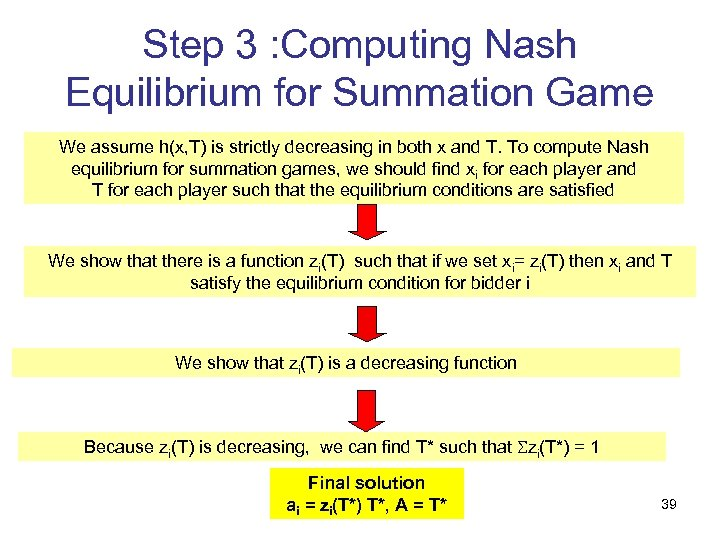Step 3 : Computing Nash Equilibrium for Summation Game We assume h(x, T) is strictly decreasing in both x and T. To compute Nash equilibrium for summation games, we should find xi for each player and T for each player such that the equilibrium conditions are satisfied We show that there is a function zi(T) such that if we set xi= zi(T) then xi and T satisfy the equilibrium condition for bidder i We show that zi(T) is a decreasing function Because zi(T) is decreasing, we can find T* such that zi(T*) = 1 Final solution ai = zi(T*) T*, A = T* 39